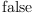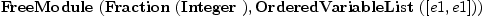login  home  contents  what's new  discussion  bug reports help  links  subscribe  changes  refresh  edit

FreeModule implements free module over a ring with generators indexed by a set. Each element can be expressed as a finite linear combination of generators. Only non-zero terms are stored.

This domain implements linear combinations of elements from the domain S with coefficients in the domain R where S is a set and R is a ring (which may be non-commutative).

A FreeModule over a [Field]? is a VectorSpace? unfortunately this is not currently understood by Axiom:

fricas
FreeModule(Fraction Integer,OrderedVariableList [e1,e1]) has VectorSpace(Fraction Integer)(1)
Type: Boolean

        if R has Field then VectorSpace(R)
...
if R has Field then
if S has Finite then
dimension():CardinalNumber == coerce size()$S else dimension():CardinalNumber == Aleph(0)  fricas F2:=FreeModule(Fraction Integer,OrderedVariableList [e1,e1])(2) Type: Type fricas F2 has VectorSpace(Fraction Integer)(3) Type: Boolean fricas dimension()$F2
The function dimension is not implemented in FreeModule(Fraction(
Integer),OrderedVariableList([e1,e1])) .

 Subject:   Be Bold !! ( 14 subscribers )